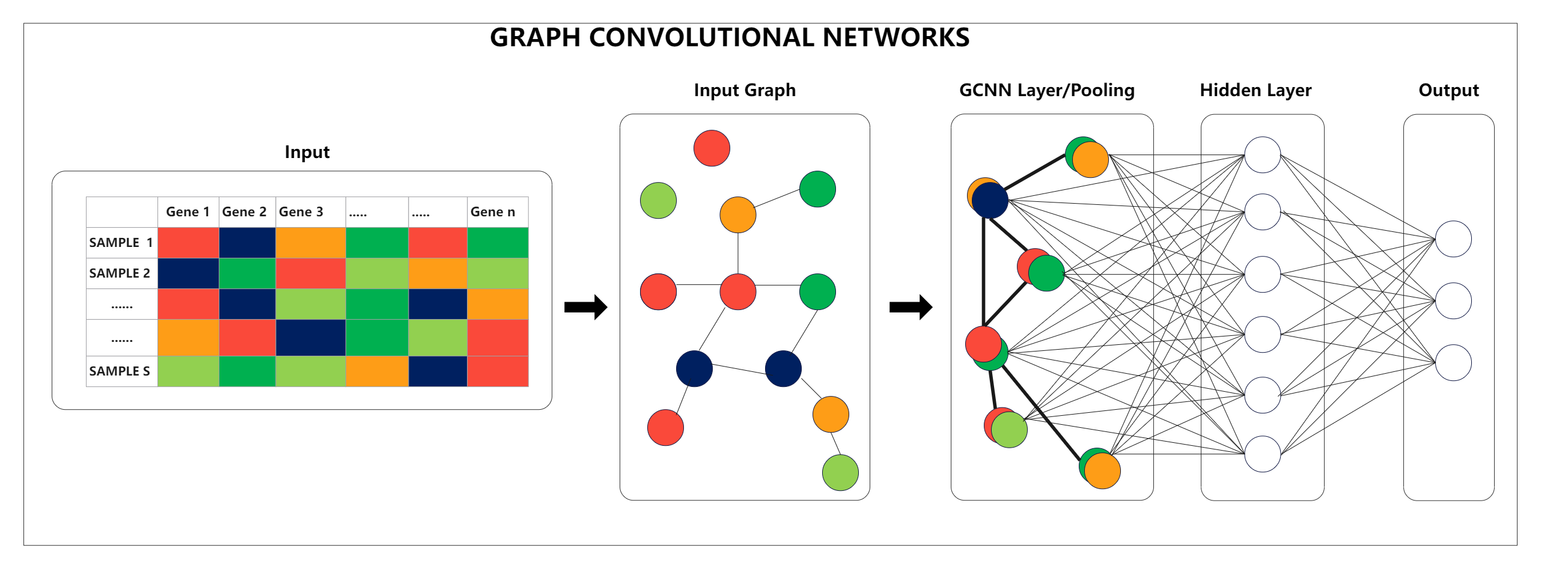Template Community / Graph Convolutional Networks

# Graph Convolutional NetworksCommunity Helper
Published on 2022-07-06
Edit OnlineThis approach for semi-supervised learning on graph-structured data is called Graph Convolutional Networks. It is based on an efficient variant of convolutional neural networks that operate on graphs directly. A localized first-order approximation of spectral graph convolutions motivates the choice of convolutional architecture. The Graph Convolutional Networks model learns hidden layer representations that encode both local graph structure and node features as the number of graph edges increases linearly. As "Convolutional" implies, the concept originated in Images and was then applied to Graphs. Graphs are much more complex when Images have a fixed structure. We obtain feature information for each node from all of its neighbors, as well as the node's feature.
Tag
Network Diagram Collection
Neural Network Diagram
Convolutional Network
Report
0
184PostRecommended Templates